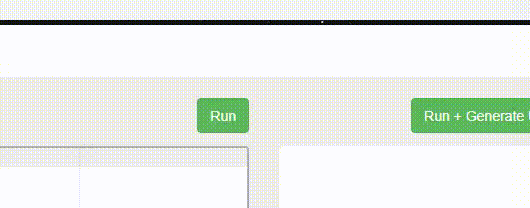Open in App
Not now

# JavaScript Index inside map() Function

• Last Updated : 26 Dec, 2022

In JavaScript, the map() method handles array elements, which creates a new array with the help of results obtained from calling function for each and every array element in an array. The index is used inside the map() method to state the position of each element in an array, but it doesn’t change the original array.

Syntax:

```array.map(function(currentelement, index, arrayobj) {

// Returns the new value instead of the item

});```

Parameters: The Index inside function accepts three parameters as mentioned above and described below:

• currentelement: The currentelement is a required argument in the map() which is the value of the current element.
• index: The index is an optional argument map() which is an array index of provided current element.
• arrayobj: The arrayobj is an optional argument in the map() which is the array object where the current element belongs.

Example 1: The below example illustrates the Index inside the map() function:

## html

 `<``body` `style``=``"text-align:center;"``> ` ` `  `    ``<``h1` `style``=``"color:green;padding:13px;"``> ` `        ``GeeksforGeeeks ` `    `` ` ` `  `    ``<``h3``>Index inside map() Function ` ` `  `    ``<``script``> ` `        ``var student = ["Arun", "Arul", ` `            ``"Sujithra", "Jenifer", "Wilson"]; ` `         `  `        ``student.map((stud, index) => { ` `            ``alert("Hello... " + stud + "\n"); ` `             `  `            ``var index = index + 1; ` `             `  `            ``alert("Your Position in Top 5" ` `                ``+ " Rank is " + index + "\n"); ` `        ``}); ` `    `` ` ``

Output:Example 2: This example prints the values and index of the array elements using the above-described approach.

## html

 `<``body` `style``=``"text-align:center;"``> ` ` `  `    ``<``h1` `style``=``"color:green;padding:13px;"``> ` `        ``GeeksforGeeeks ` `    `` ` ` `  `    ``<``h3``>Index inside map() Function ` ` `  `    ``<``script``> ` `        ``var webname = ["welcome", "to", ` `                    ``"GeeksforGeeeks"]; ` `         `  `        ``webname.map((web, index) => { ` `            ``document.write(web + "<``br``>"); ` `        ``}); ` `         `  `        ``// check in console ` `        ``webname.map((web, index)=> console.log(web, index)); ` `    `` ` ``

Output:

```welcome 0
to 1
GeeksforGeeeks 2```

Supported Browsers: The browsers supported by the Index inside map() function are listed below: